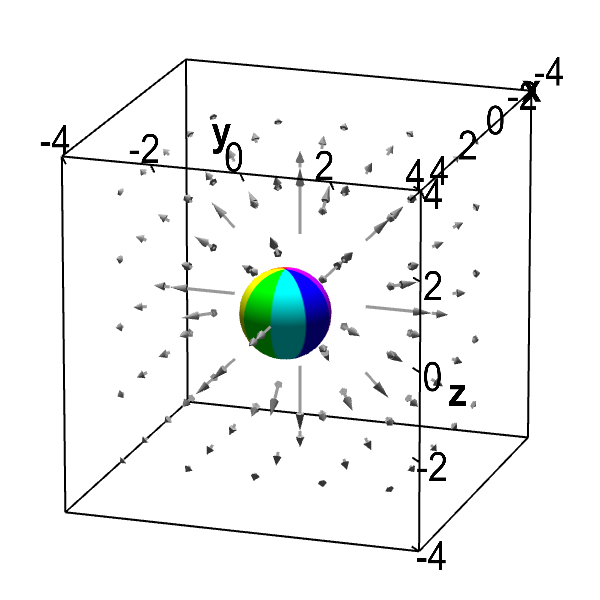# Math Insight

### Applet: Outward flowing vector field with zero divergenceThis vector field illustrates some of the subtlety of divergence. The vector field is radiating outward, so it looks like it is expanding. However, the vector field decreases in magnitude with distance from the origin just fast enough to cancel to effect of outward radiation and keep the divergence at zero. As long as the sphere is away from the origin (the applet won't allow the sphere to be moved to the origin), the net flow into the sphere is zero. The divergence is not defined at the origin, so we ignore that point.

To reconcile the zero divergence with the picture, notice that, on one hand, the flow out of the sphere is slower than the flow into the sphere, as the arrows are getting shorter. On the other hand, because the flow is radiating outward, the fluid is flowing out of the sphere across more than half of its surface. For this vector field, these two effects cancel each other out, and the net flow into the sphere is exactly equal to the net flow out of the sphere. If we stay away from the origin, the fluid is neither expanding nor compressing and the divergence is zero.

This vector field is $$\dlvf(x,y,z) = \frac{(x,y,z)}{(x^2+y^2+z^2)^{3/2}},$$ for $(x,y,z) \ne (0,0,0)$. (It is not defined at the origin.) The divergence of $\dlvf$ away from the origin is \begin{align*} \div \dlvf(x,y,z) &= \pdiff{}{x}\frac{x}{(x^2+y^2+z^2)^{3/2}} + \pdiff{}{y}\frac{y}{(x^2+y^2+z^2)^{3/2}} + \pdiff{}{z}\frac{z}{(x^2+y^2+z^2)^{3/2}} \\ &= \frac{(x^2+y^2+z^2) - 3x^2} {(x^2+y^2+z^2)^{5/2}} + \frac{(x^2+y^2+z^2) - 3y^2} {(x^2+y^2+z^2)^{5/2}} + \frac{(x^2+y^2+z^2) - 3z^2} {(x^2+y^2+z^2)^{5/2}}\\ &= \frac{3(x^2+y^2+z^2) - 3(x^2+y^2+z^2)} {(x^2+y^2+z^2)^{5/2}} =0. \end{align*}